# 分库分表之 Sharding-JDBC 中间件（下）

danielmou

0收藏

### 4. 我的特性和我的工作方法

#### 4.2. 一些核心概念

##### 4.2.4. 分片策略和分片算法

``````......
// 配置 t_order 被拆分到多个子库的策略
orderTableRuleConfig.setDatabaseShardingStrategy(
new StandardShardingStrategyConfiguration(
"user_id",
"dbShardingAlgorithm"
)
);
// 配置 t_order 被拆分到多个子表的策略
orderTableRuleConfig.setTableShardingStrategy(
new StandardShardingStrategyConfiguration(
"order_id",
"tableShardingAlgorithm"
)
);
......
ShardingRuleConfiguration shardingRuleConfig =     new ShardingRuleConfiguration();
// 配置 t_order 被拆分到多个子库的算法
Properties dbShardingAlgorithmrProps =     new Properties();
dbShardingAlgorithmrProps.setProperty(
"algorithm-expression",
"ds\${user_id % 2}"
);
shardingRuleConfig.getShardingAlgorithms().put(
"dbShardingAlgorithm",
new ShardingSphereAlgorithmConfiguration("INLINE", dbShardingAlgorithmrProps)
);
// 配置 t_order 被拆分到多个子表的算法
Properties tableShardingAlgorithmrProps =     new Properties();
tableShardingAlgorithmrProps.setProperty(
"algorithm-expression",
"t_order\${order_id % 2}"
);
shardingRuleConfig.getShardingAlgorithms().put(
"tableShardingAlgorithm",
new ShardingSphereAlgorithmConfiguration("INLINE", tableShardingAlgorithmrProps)
);
......``````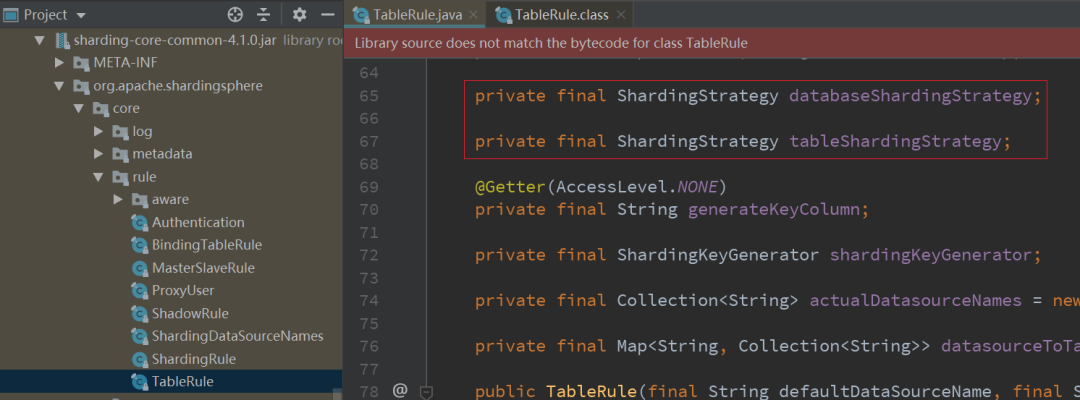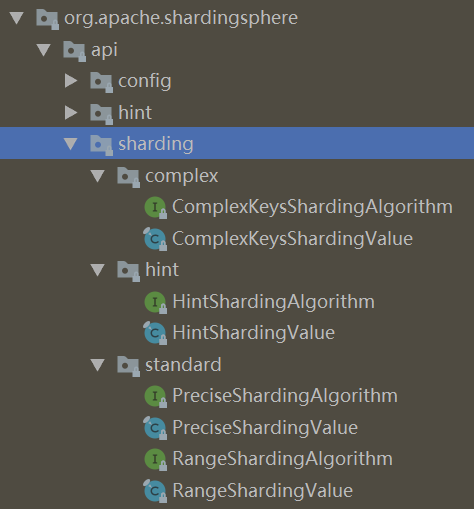``````    package org.apache.shardingsphere.core.strategy.route.standard;

......

public     final     class StandardShardingStrategy     implements ShardingStrategy {

private     final String shardingColumn;

/**
* 要配合 PreciseShardingAlgorithm 或 RangeShardingAlgorithm 使用
* 标准分片策略
*/
private     final PreciseShardingAlgorithm preciseShardingAlgorithm;

private     final RangeShardingAlgorithm rangeShardingAlgorithm;

public StandardShardingStrategy(
// 传入分片配置
final StandardShardingStrategyConfiguration standardShardingStrategyConfig
){
......

// 从配置中提取分片键
shardingColumn = standardShardingStrategyConfig.getShardingColumn();
// 从配置中提取分片算法
preciseShardingAlgorithm = standardShardingStrategyConfig.getPreciseShardingAlgorithm();
rangeShardingAlgorithm = standardShardingStrategyConfig.getRangeShardingAlgorithm();
}

@Override
public Collection<String> doSharding(
// 所有可能的分片表(或分片库)名称
final Collection<String> availableTargetNames,
// 分片键的值
final Collection<RouteValue> shardingValues,
final ConfigurationProperties properties
) {
RouteValue shardingValue = shardingValues.iterator().next();
Collection<String> shardingResult
= shardingValue     instanceof ListRouteValue
// 处理精确分片
? doSharding(availableTargetNames, (ListRouteValue) shardingValue)
// 处理范围分片
: doSharding(availableTargetNames, (RangeRouteValue) shardingValue);
Collection<String> result =     new TreeSet<>(String.CASE_INSENSITIVE_ORDER);

// 根据分片键的值，找到对应的分片表(或分片库)名称并返回
return result;
}

/**
* 处理范围分片
*/
@SuppressWarnings("unchecked")
private Collection<String> doSharding(
// 所有可能的分片表(或分片库)名称
final Collection<String> availableTargetNames,
// 分片键的值
final RangeRouteValue<?> shardingValue
) {
......
// 调用 rangeShardingAlgorithm.doSharding()根据分片键的值找到对应的
// 分片表(或分片库)名称并返回，rangeShardingAlgorithm.doSharding()
// 由你们自己实现
return rangeShardingAlgorithm.doSharding(
availableTargetNames,
new RangeShardingValue(
shardingValue.getTableName(),
shardingValue.getColumnName(),
shardingValue.getValueRange()
)
);
}

/**
* 处理精确分片
*/
@SuppressWarnings("unchecked")
private Collection<String> doSharding(
// 所有可能的分片表(或分片库)名称
final Collection<String> availableTargetNames,
// 分片键的值
final ListRouteValue<?> shardingValue
) {
for (Comparable<?> each : shardingValue.getValues()) {
// 调用 preciseShardingAlgorithm.doSharding()根据分片键的值找到对应的
// 分片表(或分片库)名称并返回，preciseShardingAlgorithm.doSharding()
// 由你们自己实现
String target
= preciseShardingAlgorithm.doSharding(
availableTargetNames,
new PreciseShardingValue(
shardingValue.getTableName(),
shardingValue.getColumnName(),
each
)
);
if (    null != target) {
}
}
return result;
}

/**
* 获取所有的分片键
*/
@Override
public Collection<String> getShardingColumns(){
Collection<String> result =     new TreeSet<>(String.CASE_INSENSITIVE_ORDER);
return result;
}
}``````

``````    package org.apache.shardingsphere.api.sharding.standard;

......

public     interface PreciseShardingAlgorithm<T     extends Comparable<?>>
extends ShardingAlgorithm {

/**
* @param 所有可能的分片表(或分片库)名称
* @param 分片键的值
* @return 根据分片键的值，找到对应的分片表(或分片库)名称并返回
*/
String doSharding(
Collection<String> availableTargetNames,
PreciseShardingValue<T> shardingValue
);
}``````

``````    package org.apache.shardingsphere.api.sharding.standard;

......

public     interface RangeShardingAlgorithm<T     extends Comparable<?>>
extends ShardingAlgorithm {

/**
* @param 所有可能的分片表(或分片库)名称
* @param 分片键的值
* @return 根据分片键的值，找到对应的分片表(或分片库)名称并返回
*/
Collection<String> doSharding(
Collection<String> availableTargetNames,
RangeShardingValue<T> shardingValue
);
}
``````

``````策略一：设置 6 个分片
t_order.order_id % 6 == 0 的查询分片到 t_order0
t_order.order_id % 6 == 1 的查询分片到 t_order1
t_order.order_id % 6 == 2 的查询分片到 t_order2
t_order.order_id % 6 == 3 的查询分片到 t_order3
t_order.order_id % 6 == 4 的查询分片到 t_order4
t_order.order_id % 6 == 5 的查询分片到 t_order5

t_order.order_id % 6     in (0,2,4) 的查询分片到 t_order1
t_order.order_id % 6     in (1,3,5) 的查询分片到 t_order1

t_order.order_id between 0 and 10000 的查询分片到 t_order0
t_order.order_id between 10000 and 20000 的查询分片到 t_order1
t_order.order_id between 20000 and 30000 的查询分片到 t_order2
t_order.order_id between 30000 and 40000 的查询分片到 t_order3
t_order.order_id between 40000 and 50000 的查询分片到 t_order4
t_order.order_id between 50000 and 60000 的查询分片到 t_order5

t_order.order_id <=10000 的查询分片到 t_order0
t_order.order_id >10000 的查询分片到 t_order1

......``````

1）分片键 order_id

2）描述以上分片策略内容的 PreciseShardingAlgorithm（接口）的实现类或 RangeShardingAlgorithm（接口）的实现类

3）前两项（即分片策略）的作用目标 t_order 表

``````-- 注：使用 t_order.order_id 作为 t_order 表的分片键
SELECT o.*     FROM t_order o     WHERE o.order_id = 10;
SELECT o.*     FROM t_order o     WHERE o.order_id     IN (10, 11);
SELECT o.*     FROM t_order o     WHERE o.order_id > 10;
SELECT o.*     FROM t_order o     WHERE o.order_id <= 11;
SELECT o.*     FROM t_order o     WHERE o.order_id     BETWEEN 10     AND 12;
......
INSERT     INTO t_order(order_id, user_id)     VALUES (20, 1001);
......
DELETE     FROM t_order o     WHERE o.order_id = 10;
DELETE     FROM t_order o     WHERE o.order_id     IN (10, 11);
DELETE     FROM t_order o     WHERE o.order_id > 10;
DELETE     FROM t_order o     WHERE o.order_id <= 11;
DELETE     FROM t_order o     WHERE o.order_id     BETWEEN 10     AND 12;
......
UPDATE t_order o     SET o.update_time =     NOW()     WHERE o.order_id = 10;
......``````

``````    package org.apache.shardingsphere.core.strategy.route.complex;

......

public     final     class ComplexShardingStrategy     implements ShardingStrategy {

@Getter
private     final Collection<String> shardingColumns;

/**
* 要配合 ComplexKeysShardingAlgorithm 使用复合分片策略
*/
private     final ComplexKeysShardingAlgorithm shardingAlgorithm;

public ComplexShardingStrategy(
// 传入分片配置
final ComplexShardingStrategyConfiguration complexShardingStrategyConfig
){
......
// 从配置中提取分片键
shardingColumns =     new TreeSet<>(String.CASE_INSENSITIVE_ORDER);
Splitter
.on(",")
.trimResults()
.splitToList(complexShardingStrategyConfig.getShardingColumns())
);
// 从配置中提取分片算法
shardingAlgorithm = complexShardingStrategyConfig.getShardingAlgorithm();
}

@SuppressWarnings("unchecked")
@Override
public Collection<String> doSharding(
// 所有可能的分片表(或分片库)名称
final Collection<String> availableTargetNames,
// 分片键的值
final Collection<RouteValue> shardingValues,
final ConfigurationProperties properties
) {
Map<String, Collection<Comparable<?>>> columnShardingValues
=     new HashMap<>(shardingValues.size(), 1);
Map<String, Range<Comparable<?>>> columnRangeValues
=     new HashMap<>(shardingValues.size(), 1);
String logicTableName = "";

// 提取多个分片键的值
for (RouteValue each : shardingValues) {
if (each     instanceof ListRouteValue) {
columnShardingValues.put(
each.getColumnName(),
((ListRouteValue) each).getValues()
);
}     else     if (each     instanceof RangeRouteValue) {
columnRangeValues.put(
each.getColumnName(),
((RangeRouteValue) each).getValueRange()
);
}
logicTableName = each.getTableName();
}
Collection<String> shardingResult
// 调用 shardingAlgorithm.doSharding()根据分片键的值找到对应的
// 分片表(或分片库)名称并返回，shardingAlgorithm.doSharding()
// 由你们自己实现
= shardingAlgorithm.doSharding(
availableTargetNames,
new ComplexKeysShardingValue(
logicTableName,
columnShardingValues,
columnRangeValues)
);
Collection<String> result =     new TreeSet<>(String.CASE_INSENSITIVE_ORDER);

// 根据分片键的值，找到对应的分片表(或分片库)名称并返回
return result;
}
}``````

``````    package org.apache.shardingsphere.api.sharding.complex;

......

public     interface ComplexKeysShardingAlgorithm<T     extends Comparable<?>>
extends ShardingAlgorithm {

/**
* @param 所有可能的分片表(或分片库)名称
* @param 分片键的值
* @return 根据分片键的值，找到对应的分片表(或分片库)名称并返回
*/
Collection<String> doSharding(
Collection<String> availableTargetNames,
ComplexKeysShardingValue<T> shardingValue
);
}``````

``````策略一：设置 4 个分片
t_order.order_id % 2 == 0 && t_order.user_id % 2 == 0 的查询分片到 t_order0
t_order.order_id % 2 == 0 && t_order.user_id % 2 == 1 的查询分片到 t_order1
t_order.order_id % 2 == 1 && t_order.user_id % 2 == 0 的查询分片到 t_order2
t_order.order_id % 2 == 1 && t_order.user_id % 2 == 1 的查询分片到 t_order3

t_order.order_id between 0 and 40000 && t_order.user_id between 0 and 500 的查询分片到 t_order0
t_order.order_id between 0 and 40000 && t_order.user_id between 500 and 1000 的查询分片到 t_order1
t_order.order_id between 40000 and 60000 && t_order.user_id between 0 and 500 的查询分片到 t_order2
t_order.order_id between 40000 and 60000 && t_order.user_id between 500 and 1000 的查询分片到 t_order3

......``````

1）分片键 order_id 和 user_id

2）描述以上分片策略内容的 ComplexKeysShardingAlgorithm（接口）的实现类

3）前两项（即分片策略）的作用目标 t_order 表

``````-- 注：使用 t_order.order_id、t_order.user_id 作为 t_order 表的分片键
SELECT o.*     FROM t_order o     WHERE o.order_id = 10;
SELECT o.*     FROM t_order o     WHERE o.order_id     IN (10, 11);
SELECT o.*     FROM t_order o     WHERE o.order_id > 10;
SELECT o.*     FROM t_order o     WHERE o.order_id <= 11;
SELECT o.*     FROM t_order o     WHERE o.order_id     BETWEEN 10     AND 12;
......
INSERT     INTO t_order(order_id, user_id)     VALUES (20, 1001);
......
DELETE     FROM t_order o     WHERE o.order_id = 10;
DELETE     FROM t_order o     WHERE o.order_id     IN (10, 11);
DELETE     FROM t_order o     WHERE o.order_id > 10;
DELETE     FROM t_order o     WHERE o.order_id <= 11;
DELETE     FROM t_order o     WHERE o.order_id     BETWEEN 10     AND 12;
......
UPDATE t_order o     SET o.update_time =     NOW()     WHERE o.order_id = 10;
......
SELECT o.*     FROM t_order o     WHERE o.order_id = 10     AND user_id = 1001;
SELECT o.*     FROM t_order o     WHERE o.order_id     IN (10, 11)     AND user_id     IN (......);
SELECT o.*     FROM t_order o     WHERE o.order_id > 10     AND user_id > 1000;
SELECT o.*     FROM t_order o     WHERE o.order_id <= 11     AND user_id <= 1000;
SELECT o.*     FROM t_order o     WHERE (o.order_id     BETWEEN 10     AND 12)     AND (o.user_id     BETWEEN 1000     AND 2000);
......
INSERT     INTO t_order(order_id, user_id)     VALUES (21, 1002);
......
DELETE     FROM t_order o     WHERE o.order_id = 10     AND user_id = 1001;
DELETE     FROM t_order o     WHERE o.order_id     IN (10, 11)     AND user_id     IN (......);
DELETE     FROM t_order o     WHERE o.order_id > 10     AND user_id > 1000;
DELETE     FROM t_order o     WHERE o.order_id <= 11     AND user_id <= 1000;
DELETE     FROM t_order o     WHERE (o.order_id     BETWEEN 10     AND 12)     AND (o.user_id     BETWEEN 1000     AND 2000);
......
UPDATE t_order o     SET o.update_time =     NOW()     WHERE o.order_id = 10     AND user_id = 1001;
......``````

Hint 分片策略对应 HintShardingStrategy 这个 final class，同标准分片策略和符合分片策略的代码类似，HintShardingStrategy 中包含一个 HintShardingAlgorithm 接口的实例，并调用它的 doSharding()方法。你们要自己去实现这个 HintShardingAlgorithm 接口中的 doSharding()方法，这样我就能知道如何根据分片键的值，找到对应的分片表(或分片库)。此处不在展示 HintShardingStrategy 和 HintShardingAlgorithm 的源码。

Hint 分片策略是指通过 Hint 指定分片值而非从 SQL 中提取分片值的方式进行分片的策略。简单来讲就是我收到的 SQL 语句中不包含分片值（像上面给出的几段 SQL 就是包含分片值的 SQL），但是工程师会通过我提供的 Java API 将分片值暗示给我，这样我就知道怎样路由 SQL 查询到具体的分片了。就像下面这样：

``````String sql = "SELECT * FROM t_order";
try (
// HintManager 是使用“暗示”的工具，它会把暗示的分片值放入
// 时候就能获取到分片值
HintManager hintManager = HintManager.getInstance();
Connection conn = dataSource.getConnection();
PreparedStatement pstmt = conn.prepareStatement(sql);
) {
hintManager.setDatabaseShardingValue(3);
try (ResultSet rs = pstmt.executeQuery()) {
// 若 t_order 仅仅使用 order_id 作为分片键，则这里根据暗
// 示获取了分片值，因此上面的 SQL 的实际执行效果相当于：
// SELECT * FROM t_order where order_id = 3
while (rs.next()) {
//...
}
}
}``````

``````    package org.apache.shardingsphere.core.strategy.route.none;

......

@Getter
public     final     class NoneShardingStrategy     implements ShardingStrategy {

private     final Collection<String> shardingColumns = Collections.emptyList();

@Override
public Collection<String> doSharding(
// 所有可能的分片表(或分片库)名称
final Collection<String> availableTargetNames,
// 分片键的值
final Collection<RouteValue> shardingValues,
final ConfigurationProperties properties
) {

// 不需要任何算法，不进行任何逻辑处理，直接返回
// 所有可能的分片表(或分片库)名称
return availableTargetNames;
}
}``````

Inline 分片策略，也叫做行表达式分片策略。Inline 分片策略对应 InlineShardingStrategy。Inline 分片策略是为用 Grovvy 表达式描述的分片算法准备的分片策略。文章开始展示的两段配置中就使用了 Inline 分片策略。InlineShardingStrategy 把 Grovvy 表达式当做分片算法的实现，因此 HintShardingStrategy 中不包含算法域变量，这一点有别于 StandardShardingStrategy 等 final class。这里不再展示 InlineShardingStrategy 的源码。

##### 4.2.5. 绑定表

``    SELECT i.*     FROM t_order o     JOIN t_order_item i     ON o.order_id=i.order_id     WHERE o.order_id     IN (10, 11);``

``````    SELECT i.*     FROM t_order0 o     JOIN t_order_item0 i     ON o.order_id=i.order_id     WHERE o.order_id     IN (10, 11);
SELECT i.*     FROM t_order0 o     JOIN t_order_item1 i     ON o.order_id=i.order_id     WHERE o.order_id     IN (10, 11);
SELECT i.*     FROM t_order1 o     JOIN t_order_item0 i     ON o.order_id=i.order_id     WHERE o.order_id     IN (10, 11);
SELECT i.*     FROM t_order1 o     JOIN t_order_item1 i     ON o.order_id=i.order_id     WHERE o.order_id     IN (10, 11);``````

``````    SELECT i.*     FROM t_order0 o     JOIN t_order_item0 i     ON o.order_id=i.order_id     WHERE o.order_id     IN (10, 11);
SELECT i.*     FROM t_order1 o     JOIN t_order_item1 i     ON o.order_id=i.order_id     WHERE o.order_id     IN (10, 11);``````

``````# 设置绑定表
sharding.jdbc.config.sharding.binding-tables=t_order, t_order_item``````

#### 4.3. 我处理 SQL 的过程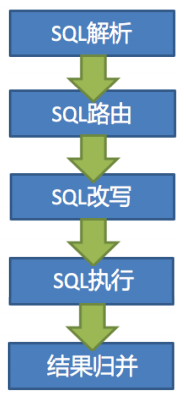1）SQL 解析（由我内核中的解析引擎完成）：先通过词法解析器将逻辑 SQL 拆分为一个个不可再分的单词，再使用语法解析器对 SQL 进行理解，并最终提炼出解析上下文。

2）SQL 路由（由我内核中的路由引擎完成）：根据解析上下文匹配用户配置的分片策略（关于用户配置的分片策略，我后文会慢慢解释），并生成路由路径，路由路径指示了 SQL 最终要到哪些分片去执行。

3）SQL 改写（由我内核中的改写引擎完成）：将 面向逻辑表 SQL 改写为在真实数据库中可以正确执行的语句（逻辑 SQL 到物理 SQL 的映射）。

4）SQL 执行（由我内核中的执行引擎完成）：通过多线程执行器异步执行路由和改写之后得到的 SQL 语句。

5）结果归并（由我内核中的归并引擎完成）：将多个执行结果集归并以便于通过统一的 JDBC 接口输出。

##### 4.3.1. SQL 解析

SQL 解析 SQL 解析分为词法解析和语法解析。

``    SELECT     id,     name     FROM t_user     WHERE     status = 'ACTIVE'     AND age > 18``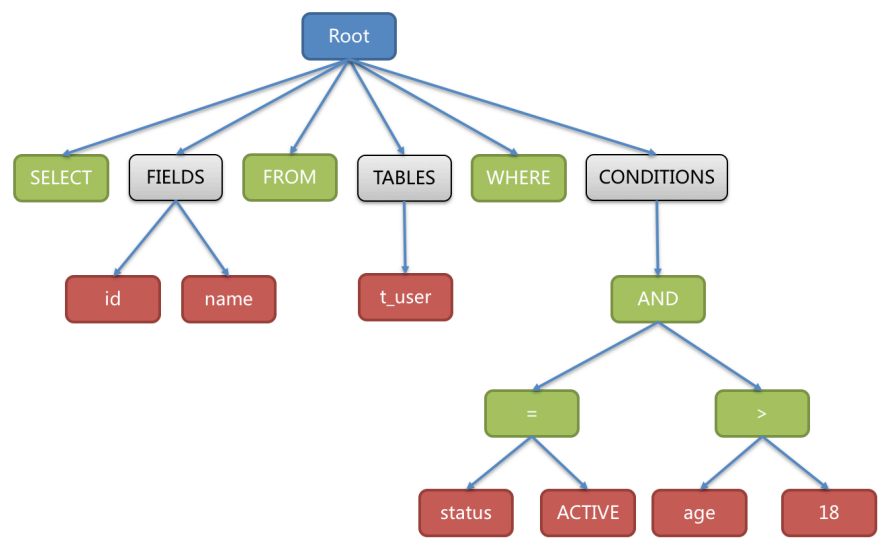SQL 解析是下面的路由、改写、执行和归并的基础。

##### 4.3.2. SQL 路由

``````-- 若仅以 user_id 作为分片键对 t_user 进行分片，且分片算法为 user_id % 5，则以下 SQL 在一个数据源内会针对一个特定分片执行：
SELECT *     FROM t_user     WHERE user_id = 1009 --路由到 t_user4 执行

-- 若仅以 user_id 作为分片键对 t_user 进行分片，且分片算法为 user_id % 5，则以下 SQL 在一个数据源内会针对多个分片执行：
SELECT *     FROM t_user     WHERE user_id     in (1002, 1003, 1009) --路由到 t_user2、t_user3、t_user4
SELECT *     FROM t_user     WHERE user_id > 1002     AND user_id <= 1004 --路由到 t_user3、t_user4
SELECT *     FROM t_user     WHERE user_id     between 1002     and 1004 --路由到 t_user2、t_user3

-- 若仅以 user_id 作为分片键对 t_user 进行分片，且分片算法为 user_id % 5，则以下 SQL 在一个数据源内会针对所有的分片执行：
SELECT     count(1)     FROM t_user --路由到 t_user0、t_user1、t_user2、t_user3、t_user4
SELECT *     FROM t_user     where age < 18 --路由到 t_user0、t_user1、t_user2、t_user3、t_user4``````
##### 4.3.3. SQL 改写

⼯程师交给我处理的 SQL 是面向逻辑表书写的 SQL，并不能够直接在数据库中执行，所以我的内核要完成 SQL 改写，将面向逻辑表的 SQL 改写面向物理表的 SQL。SQL 改写分为标识符改写、补列、分页修正、批量拆分。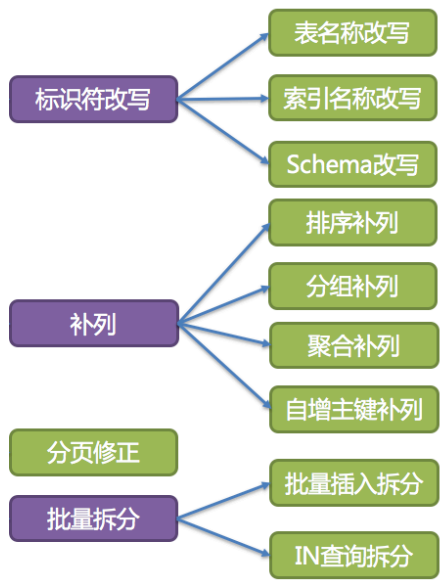1）表名称改写

``    SELECT order_id     FROM t_order     WHERE order_id=1;``

``    SELECT order_id     FROM t_order1     WHERE order_id=1;``

``    SELECT t_order.order_id     FROM t_order     AS t_order     WHERE t_order.order_id=1     AND remarks='备注 t_order xxx';``

SQL 改写则仅需要改写表名称就可以了，别名“t_order”、备注字段内容“t_order”均无需改写：

``    SELECT t_order.order_id     FROM t_order_1     AS t_order     WHERE t_order.order_id=1     AND remarks='备注 t_order xxx';``

2）索引名称改写

3）Schema 名称改写

``    SHOW     COLUMNS     FROM t_order     FROM order_ds;``

1）排序补列

``    SELECT order_id, user_id     FROM t_order     ORDER     BY user_id;``

``    SELECT order_id     FROM t_order0     ORDER     BY user_id;``

``    SELECT order_id, user_id     FROM t_order0     ORDER     BY user_id;``

``````-- 补列前（结果集 o.* 中不包含排序键 order_item_id）
SELECT o.*     FROM t_order o, t_order_item i     WHERE o.order_id=i.order_id     ORDER     BY user_id, order_item_id;

-- 补列后（结果集 o.* 中包含排序键 order_item_id）
SELECT o.*, order_item_id     FROM t_order o, t_order_item i     WHERE o.order_id=i.order_id     ORDER     BY user_id, order_item_id;``````

2）分组补列

``````-- 补列前（结果集 order_id 中不包含分组键 user_id）
SELECT order_id     FROM t_order     GROUP     BY user_id

-- 补列后（结果集 order_id 中包含分组键 user_id）
SELECT order_id, user_id     FROM t_order     GROUP     BY user_id``````

3）聚合补列

``    SELECT     AVG(age)     FROM t_user     WHERE age>=18;``

``    SELECT     COUNT(age)     AS AVG_DERIVED_COUNT,     SUM(age)     AS AVG_DERIVED_SUM     FROM t_user     WHERE age>=18;``

``````    SELECT     COUNT(age)     AS AVG_DERIVED_COUNT,     SUM(age)     AS AVG_DERIVED_SUM     FROM t_user0     WHERE age>=18;

SELECT     COUNT(age)     AS AVG_DERIVED_COUNT,     SUM(age)     AS AVG_DERIVED_SUM     FROM t_user1     WHERE age>=18;

SELECT     COUNT(age)     AS AVG_DERIVED_COUNT,     SUM(age)     AS AVG_DERIVED_SUM     FROM t_user2     WHERE age>=18;``````

``````(
四（1）班总分 +
四（2）班总分 +
四（3）班总分 +
四（4）班总分
) / (
四（1）班人数 +
四（2）班人数 +
四（3）班人数 +
四（4）班人数
)``````

``````(
四（1）班平均分 +
四（2）班平均分 +
四（3）班平均分 +
四（4）班平均分
) / 4``````

4）自增主键补列

INSERT 语句如果使用数据库自增主键，是无需写入主键字段的，依靠数据库实例本身自动产生自增主键。但单个数据库实例产生的自增主键是无法满足数据表多分片场景下的主键的唯一性要求的，因此我提供了分布式自增主键的生成算法（如雪花算法），并且可以通过补列，让使用方无需改动现有代码，即可将数据库现有的自增主键透明地替换成分布式自增主键。举例说明，假设表 t_order 的主键是 order_id，原始的 SQL 为：

``    INSERT     INTO t_example (`field1`, `field2`)     VALUES (10, 1);``

``    INSERT     INTO t_example (    id, `field1`, `field2`)     VALUES (snow_flake_id, 10, 1);``

``    SELECT age     FROM t_user     ORDER     BY age     DESC     LIMIT 1, 2;``

``````    SELECT age     FROM t_user0     ORDER     BY age     DESC     LIMIT 1, 2;
SELECT age     FROM t_user1     ORDER     BY age     DESC     LIMIT 1, 2;``````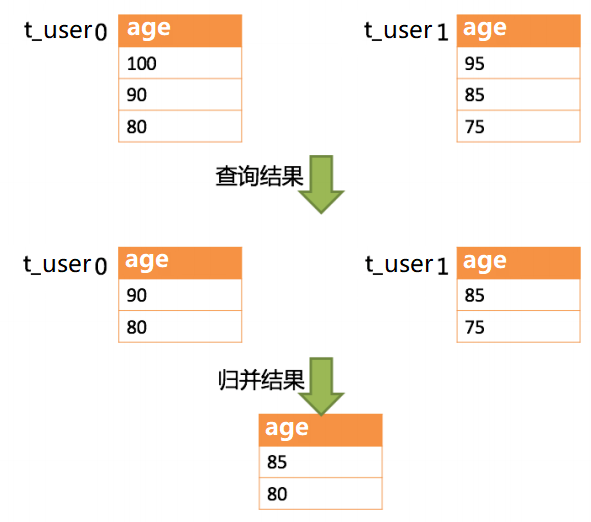``    SELECT age     FROM t_user     ORDER     BY age     DESC     LIMIT 0, 3;``

``````    SELECT age     FROM t_user0     ORDER     BY age     DESC     LIMIT 0, 3;
SELECT age     FROM t_user1     ORDER     BY age     DESC     LIMIT 0, 3;``````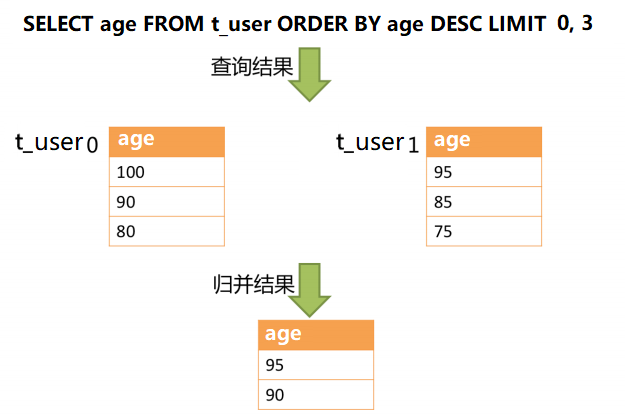1）批量插入拆分

``    INSERT     INTO t_order (order_id, xxx)     VALUES (1, 'xxx'), (2, 'xxx'), (3, 'xxx');``

``````    INSERT     INTO t_order0 (order_id, xxx)     VALUES (2, 'xxx');
INSERT     INTO t_order1 (order_id, xxx)     VALUES (1, 'xxx'), (3, 'xxx');``````

2）In 查询拆分

``    SELECT *     FROM t_order     WHERE order_id     IN (1, 2, 3);``

``````    SELECT *     FROM t_order0     WHERE order_id     IN (1, 2, 3);
SELECT *     FROM t_order1     WHERE order_id     IN (1, 2, 3);``````

``````    SELECT *     FROM t_order0     WHERE order_id     IN (2);
SELECT *     FROM t_order1     WHERE order_id     IN (1, 3);``````

##### 4.3.4. SQL 执行

1）先将 SQL 的路由结果按照数据源的名称进行分组；

2）然后通过下图的公式，可以获得每个数据库实例在 maxConnectionSizePerQuery 的允许范围内，每个连接需要执行的 SQL 路由结果组，并计算出本次请求的最优连接模式。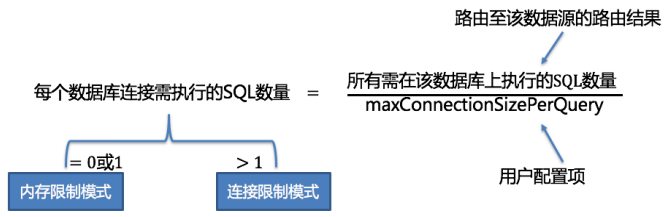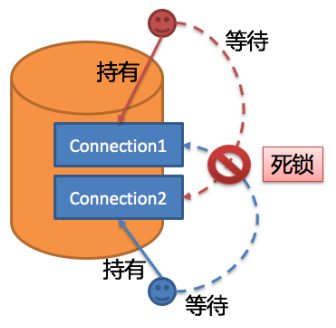1）避免锁定一次性只需要获取 1 个数据库连接的操作。因为每次仅需要获取 1 个连接，则不会发生两个请求相互等待的场景，无需锁定。对于大部分 OLTP 的操作，都是使用分片键路由至唯一的数据节点，这会使得系统变为完全无锁的状态，进一步提升了并发效率。

2）仅针对内存限制模式时才进行资源锁定。在使用连接限制模式时，所有的查询结果集将在装载至内存之后释放掉数据库连接资源，因此不会产生死锁等待的问题。

1）分组执行：分组执行将准备执行阶段生成的执行单元分组下发至我的底层执行引擎，并针对执行过程中的每个关键步骤发送事件。如：执行开始事件、执行成功事件以及执行失败事件。我的执行引擎仅关注事件的发送，它并不关心事件的订阅者。我的其他模块，如：分布式事务、调用链路追踪等，会订阅感兴趣的事件，并进行相应的处理。我通过在执行准备阶段的获取的连接模式，生成内存查询结果集或流式查询结果集，并将其传递至结果归并引擎，以进行下一步的⼯作。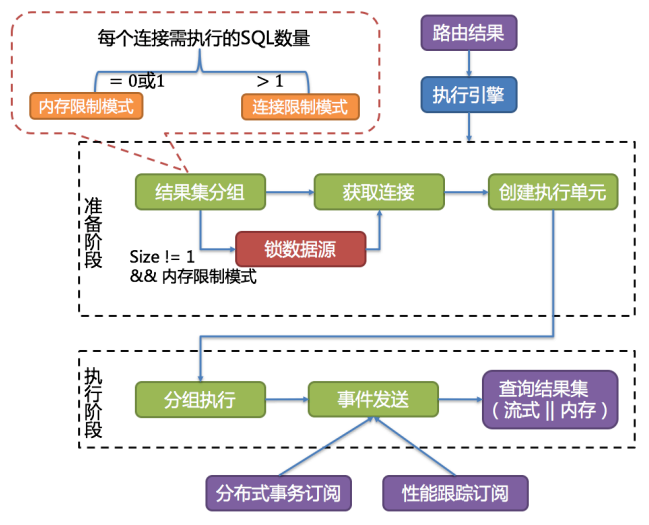2）归并结果集：请看下一节。

##### 4.3.5. 结果归并

1）流式归并

2）内存归并

3）装饰者归并

1）遍历归并

``    SELECT age     FROM t_user     where age < 18``

``````    SELECT age     FROM t_user0     where age < 18
SELECT age     FROM t_user1     where age < 18
SELECT age     FROM t_user2     where age < 18``````

2）排序归并

``    SELECT age     FROM t_user     order     by age     DESC``

``````    SELECT age     FROM t_user0     order     by age     DESC
SELECT age     FROM t_user1     order     by age     DESC
SELECT age     FROM t_user2     order     by age     DESC``````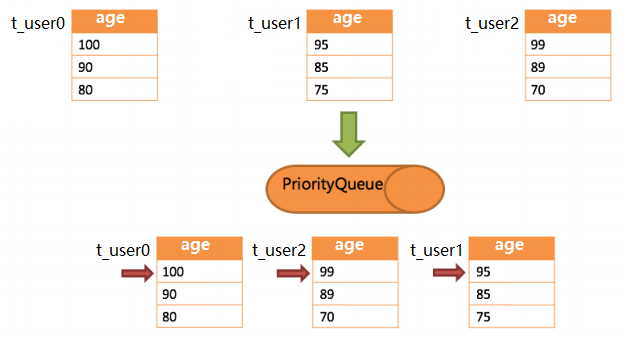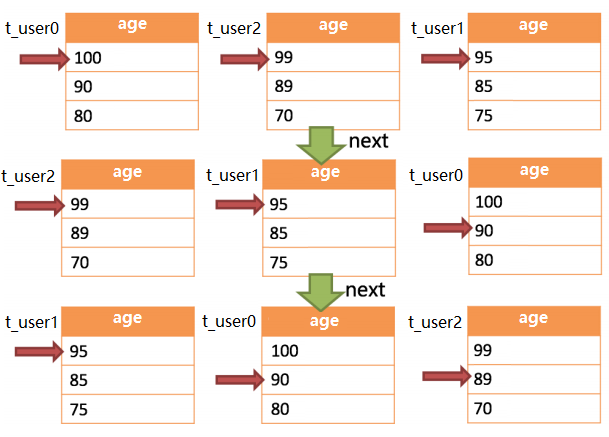3）分组归并

``    SELECT     name,     SUM(score)     as sum_score     FROM t_score     GROUP     BY     name     ORDER     BY     name     asc;``

``````    SELECT     name,     SUM(score)     as sum_score     FROM t_score_java     GROUP     BY     name     ORDER     BY     name     asc;
SELECT     name,     SUM(score)     as sum_score     FROM t_score_go     GROUP     BY     name     ORDER     BY     name     asc;
SELECT     name,     SUM(score)     as sum_score     FROM t_score_python     GROUP     BY     name     ORDER     BY     name     asc;``````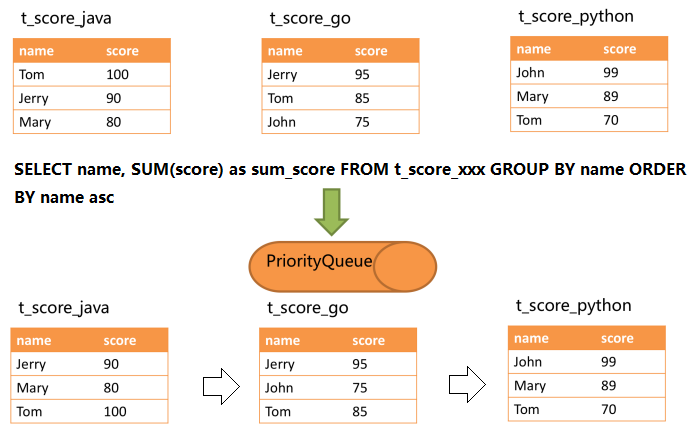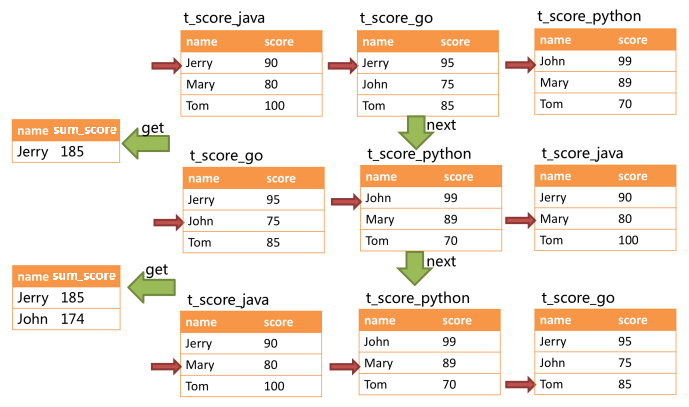``    SELECT     name,     SUM(score)     as sum_score     FROM t_score     GROUP     BY     name     ORDER     BY score     DESC;``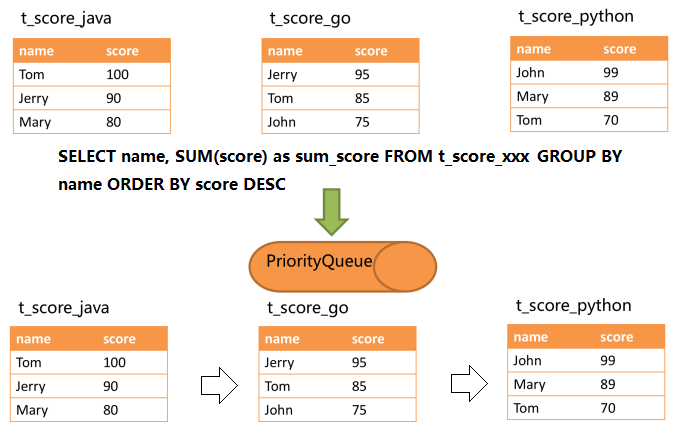4）聚合归并

``    SELECT     name,     MAX(score)     FROM t_score     GROUP     BY     name;``

``````--当 SQL 中只包含分组语句时，我会通过 SQL 改写，自动增加与分组项一致的排序项，这能够使得这句 SQL 的归并阶段从消耗内存的内存分组归并方式转化为流式分组归并方式
SELECT     name,     MAX(score)     as max_score     FROM t_score_java     GROUP     BY     name     ORDER     BY     name     ASC;
SELECT     name,     MAX(score)     as max_score     FROM t_score_go     GROUP     BY     name     ORDER     BY     name     ASC;
SELECT     name,     MAX(score)     as max_score     FROM t_score_python     GROUP     BY     name     ORDER     BY     name     ASC;``````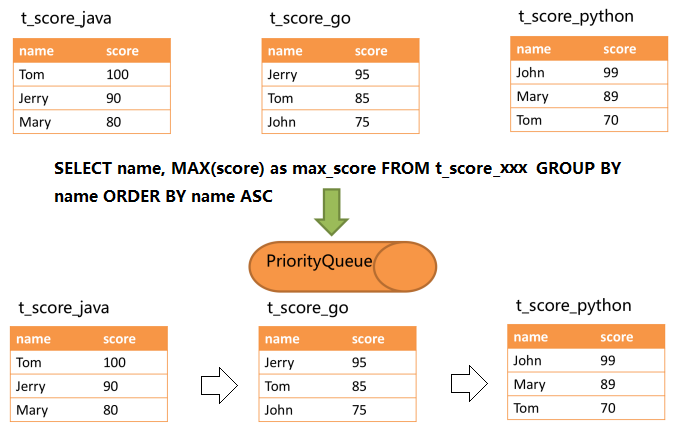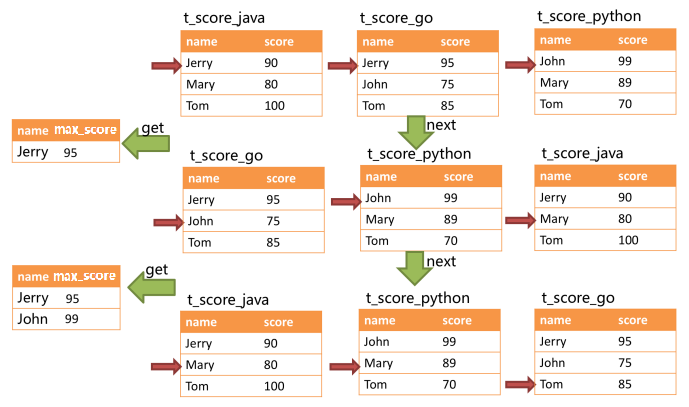5）分页归并

``    SELECT *     FROM t_order     WHERE     id > 100000     AND     id <= 100010     ORDER     BY     id;``

``    SELECT *     FROM t_order     WHERE     id > 10000000     LIMIT 10;``

### 5. 结束语

``````/**
* 所有的分片算法 interface 都包含该方法
*
* @param 所有可能的分片表(或分片库)名称
* @param 分片键的值
* @return 根据分片键的值，找到对应的分片表(或分片库)名称并返回
*/
Collection<String> doSharding(
Collection<String> availableTargetNames,
ComplexKeysShardingValue<T> shardingValue
);``````帖子
视频
声望
粉丝
社区精华内容